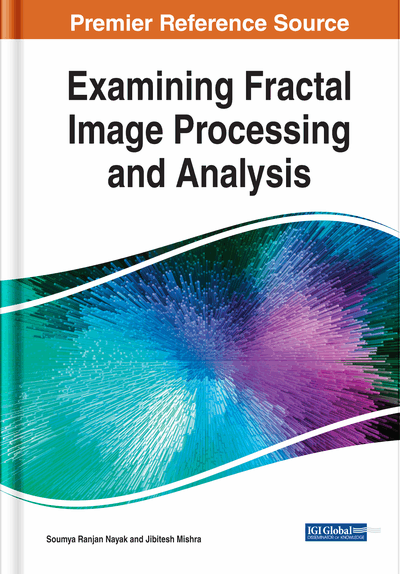# A Review of Contemporary Image Compression Techniques and Standards

Tawheed Jan Shah, M. Tariq Banday
DOI: 10.4018/978-1-7998-0066-8.ch006
OnDemand:
(Individual Chapters)
Available
\$37.50
No Current Special Offers

## Abstract

Uncompressed multimedia data such as images require huge storage space, processing power, transmission time, and bandwidth. In order to reduce the storage space, transmission time, and bandwidth, the uncompressed image data is compressed before its storage or transmission. This process not only permits a large number of images to be stored in a specified amount of storage space but also reduces the time required for them to be sent or download from the internet. In this chapter, the classification of an image on the basis of number of bits used to represent each pixel of the digital image and different types of image redundancies is presented. This chapter also introduced image compression and its classification into different lossless and lossy compression techniques along with their advantages and disadvantages. Further, discrete cosine transform, its properties, and the application of discrete cosine transform-based image compression method (i.e., JPEG compression model) along with its limitations are also discussed in detail.
Chapter Preview
Top

## Introduction

### Images and Their Classification

Image is a visual representation of a subject usually a physical object. It may be two-dimensional(2-D) or three-dimensional(3-D) such as a statue and a photograph respectively. An image is actually a rectangular grid (Rao & Hwang, 1996) of basic picture element called dot or pixel arranged in M rows and N columns, originating from the upper left corner. The expression M x N is known as the resolution of the image. Each pixel in an image has a fixed size on a given display. Mathematically, “An image may be defined as a 2-D function f(x, y), where ‘f’ is the amplitude and (x, y) are spatial co-ordinates. The amplitude ‘f’ at any pair of co-ordinates (x, y) is called the intensity value of an image (Penebaker, & Mitchell, 1993). When the intensity and co-ordinate values are finite discrete quantities, the image is called as the “Digital Image”. Since digital image is a regular arrangement of pixels so there exist certain relationships between them. Consider two pixels P(m, n) and Q(u, v) at co-ordinates (m, n) and (u,v) respectively. The distance between these two pixels is measured in order to find out how close these two pixels are and how they are related to each other. However, there are a number of ways to measure this distance, e.g. Euclidean distance, Chessboard distance and City-block distance. The Mathematical formula for calculating each of these distances is given as follows.

The Euclidean distance between two 2-D image points P(m, n) and Q(u, v) is defined as:

(1)

The Chessboard distance between two 2-D image points P(m, n) and Q(u, v) can be calculated as follows:

max(|m‑n|, |n‑v|)(2)

For the above two 2-D pixels, the City-block distance is:

|m‑u| + |n‑v|(3)

On the basis of number of bits used to represent each pixel of the digital image, these can be classified into three categories namely i) binary image, ii) grey scale Image, and iii) RGB or color image. Binary images are also called as Black and white images. This means each pixel is represented by a single bit. Since the number of bits per pixel i.e. n=1 in case of binary images, therefore the number of possible colors used to represent the image is 2n =21= 2. Typically, two colors used are black (0) and white (1 or 255) though any two colors can be used. A binary image is usually stored in computer memory as Bitmap. In grayscale images, 8-bits are used to represent each pixel (Rao & Yip, 1990). Therefore, the number of possible shades of gray is 256 ranging from 0 to 255. A pixel value of ‘0’ and ‘255’ represents the black and white color respectively. The values in between 0 to 255 are used for varying shades of gray. An RGB image is typically represented using 24 bits, 8 for red, 8 for green and 8 for blue. A 24-bit image thus yields a potential of 16.7 million color values. RGB images do not use a palette but requires three times more memory than black and white version of the same image with same size.

## Key Terms in this Chapter

MRI: Magnetic Resonance Images

MPEG: Motion Picture Experts Group

IFT: Iterated Function Theory

VQ: Vector Quantization

HVS: Human Visual System

RLC: Run Length Coding

PSNR: Peak Signal to Noise Ratio

RD: Data Redundancy

MSE: Mean Square Error

JPEG: Joint Photographic Experts Group

MRCM: Multiple Reduction Copying Machine

SQ: Scalar Quantization

DCT: Discrete Cosine Transform